Published: May 08, 2018, Edited by: Nicolas Padfield

# Rapid prototyping with Arduino and laser cutting in 4 hours

This is a new course and an in progress tutorial

For the Rapid Prototyping (short, 4 hours) course for Akademiet for Talentfulde Unge, you get

• An introduction to Arduino programming.
• A kit consisting of an Arduino microcontroller, some Neopixels, a servo, a microswitch and a potmeter
• An introduction to 2d vector drawing on computer
• An introduction to laser cutting

Then you can build some simple prototype using cardboard or HDF, the sensors in the kit, and programming.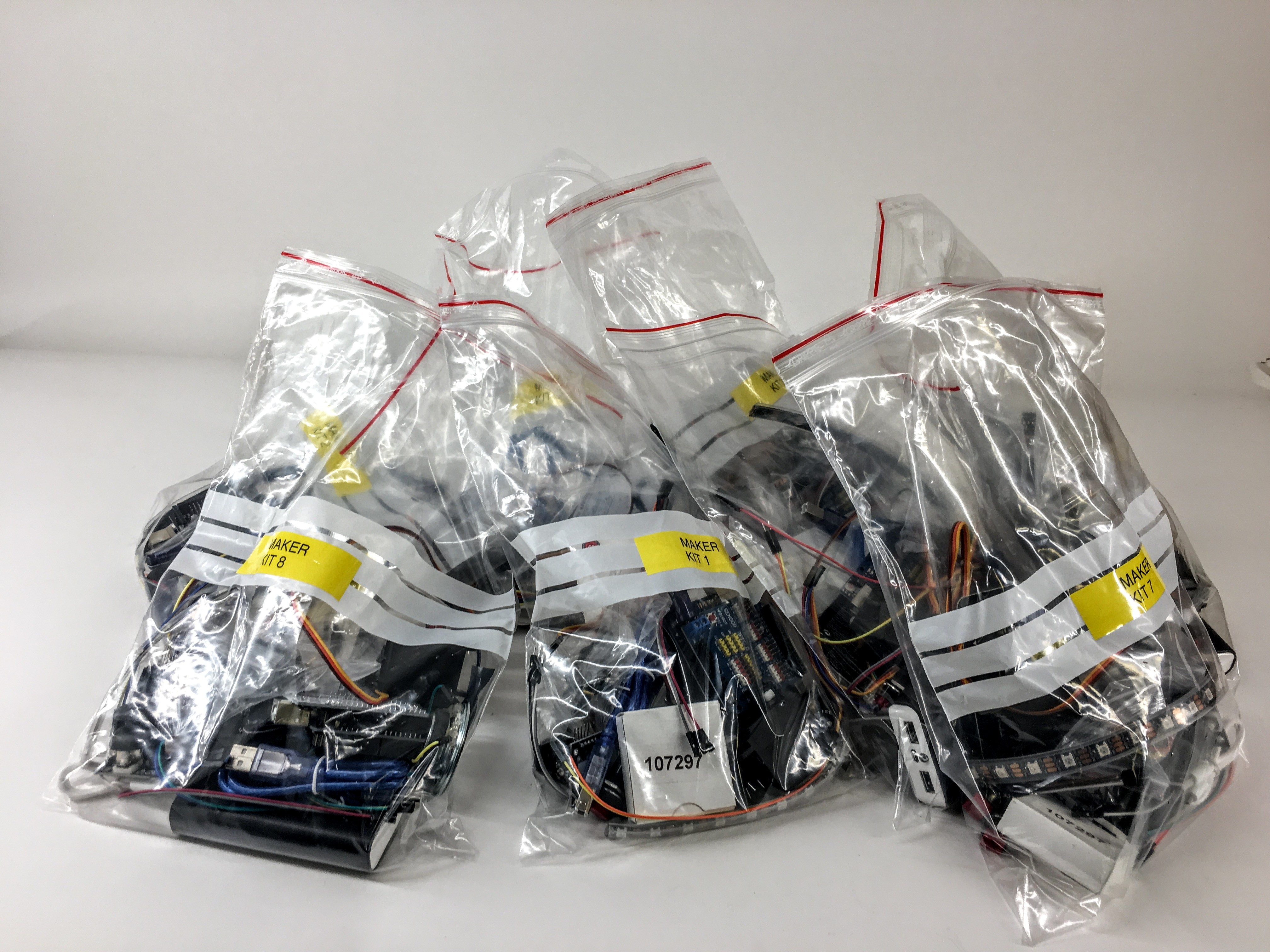## Detecting button pressed STATE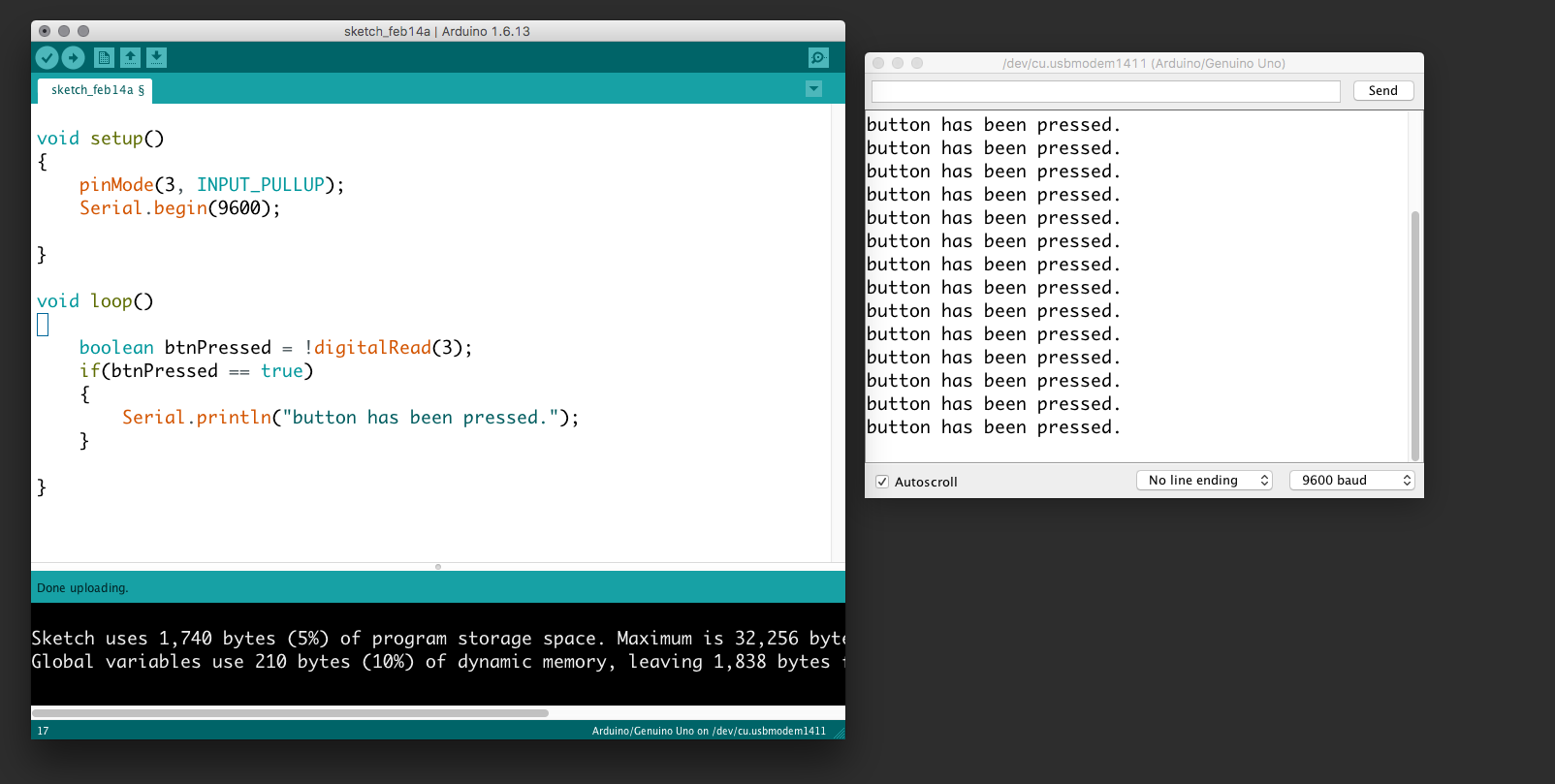In the following example, we connect a button to the Arduino and send a message repeatedly somebody presses it. Be aware that we use the internal pull-up so the button has to be connected to ground (not to +5V). Further, we use the serial communication to receive the messages to our prompt. Use the "search icon" (upper right corner in the Arduino development environment) to view the serial prompt.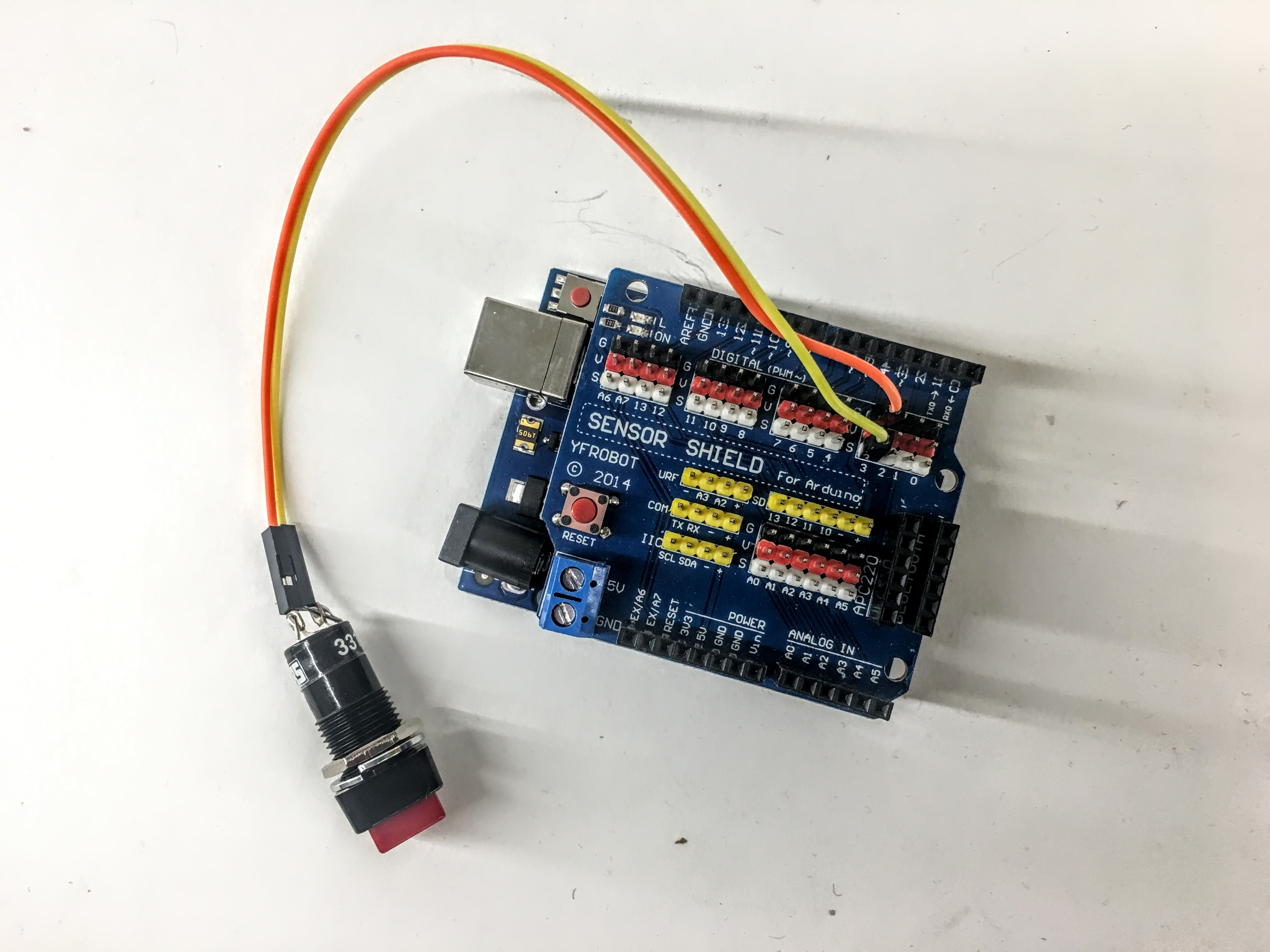``````        void setup()
{
pinMode(3, INPUT_PULLUP);
Serial.begin(9600);

}

void loop()
{
if(btnPressed == true)
{
Serial.println("button has been pressed.");
}

}
``````

## Detecting button single press - An EVENT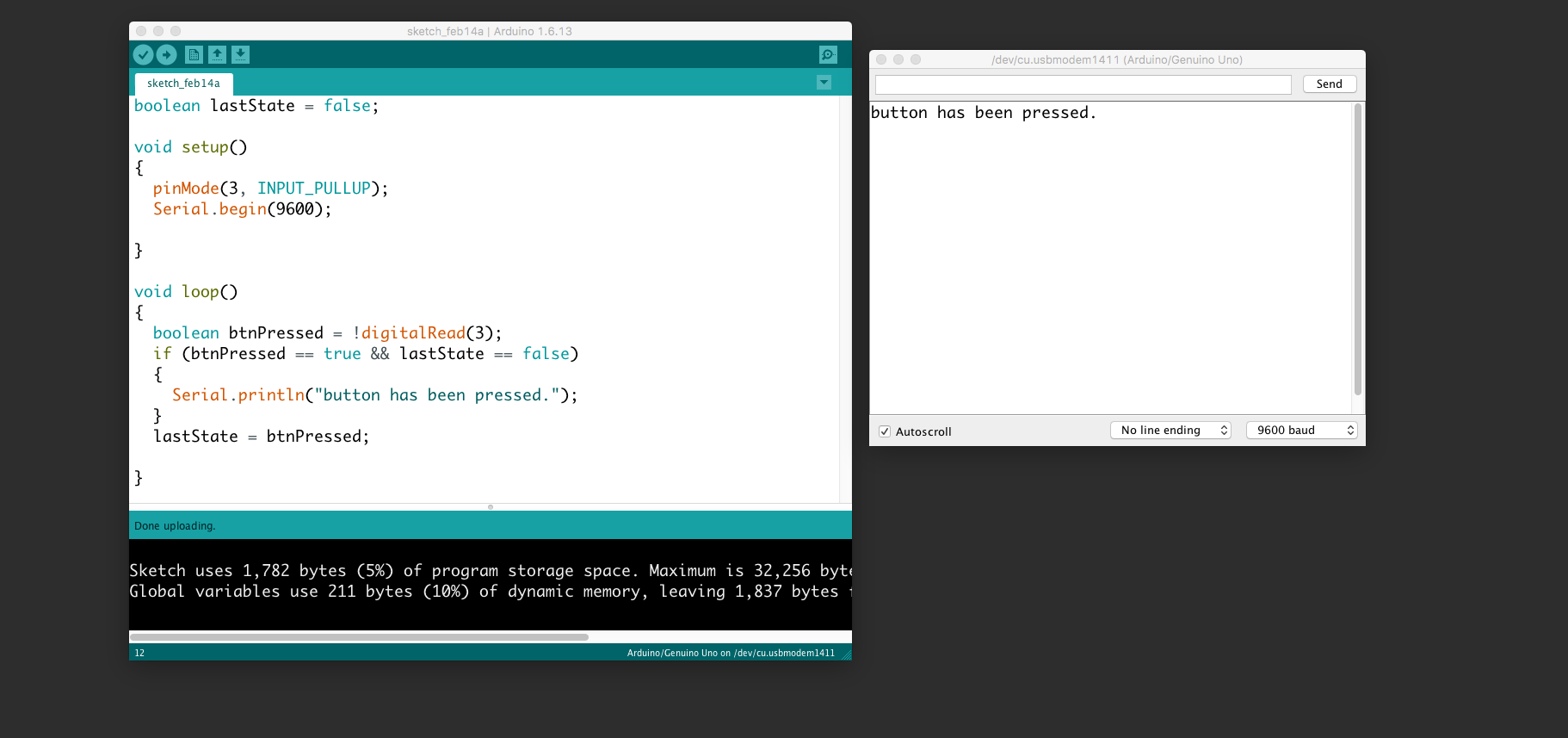The event of pressing the button. Use the same wiring as above.

``````  boolean lastState = false;

void setup()
{
pinMode(3, INPUT_PULLUP);
Serial.begin(9600);

}

void loop()
{
if (btnPressed == true && lastState == false)
{
Serial.println("button has been pressed.");
}
lastState = btnPressed;

}
``````

## Control neopixels

Neopixels are versatile light strings where each diode can be controlled individually. Each one has Red, Green, and Blue and can be from 0 to 255 in brightness. Multiple libraries exists to use noepixels. We suggest you use fastled.Search for "fastled" and click install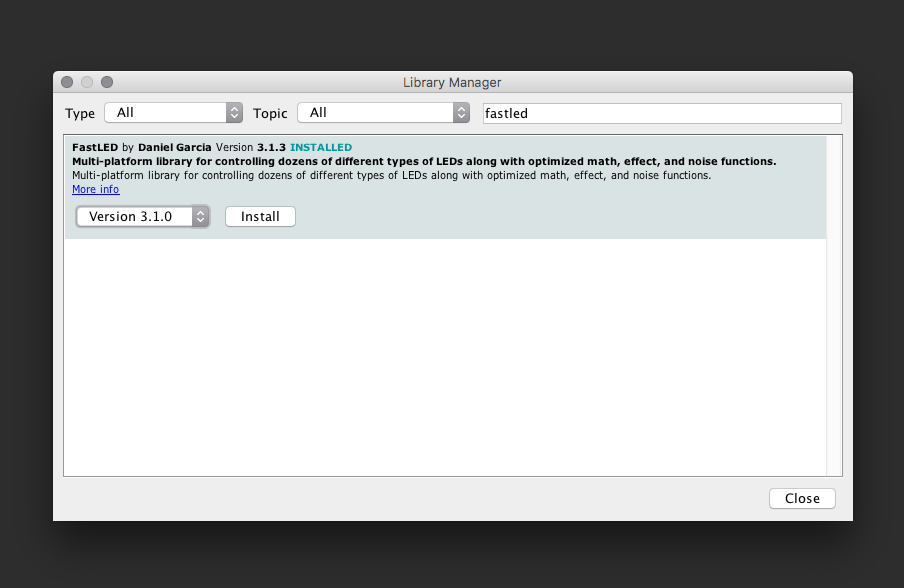Use this wiring: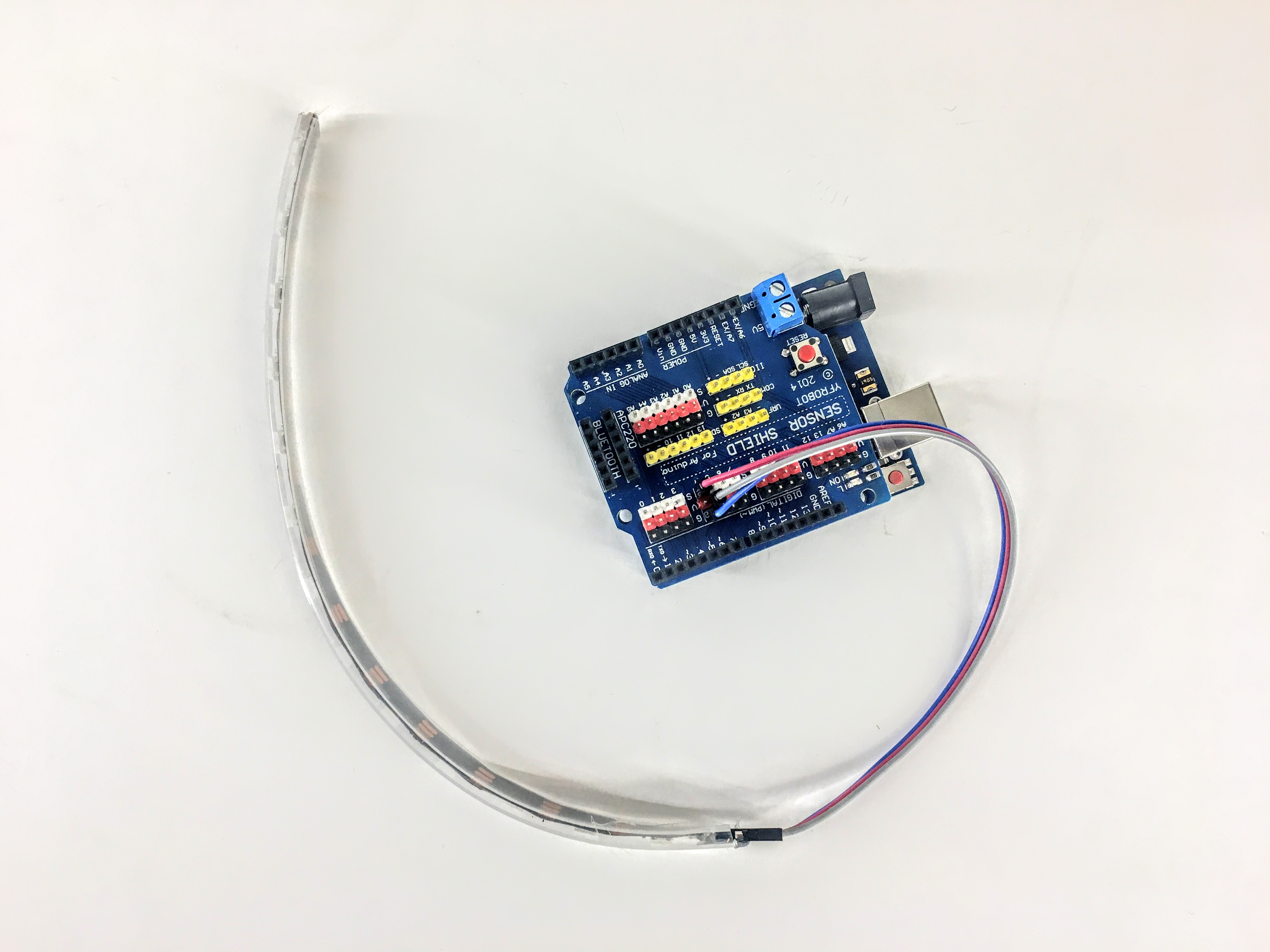IMPORTANT: connect 5v to v, Di to s and G to g AND make sure the direction is correct: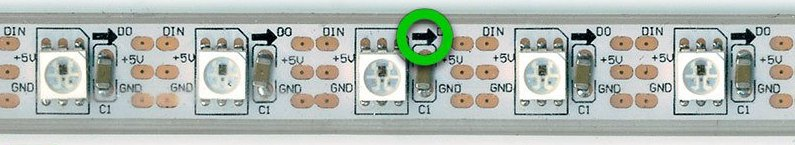This example sketch will turn red, green and blue for the first three pixels:

``````  #include "FastLED.h"

// How many leds in your strip?
#define NUM_LEDS 10
#define DATA_PIN 4

// Define the array of leds
CRGB leds[NUM_LEDS];

void setup() {
}

void loop() {
// Turn the LED on, then pause
leds= CRGB( 255, 0, 0);
leds= CRGB( 0, 255, 0);
leds= CRGB( 0, 0, 255);
FastLED.show();

}
``````

This example will turn all the 12 leds red:

``````#include "FastLED.h"

// How many leds in your strip?
#define NUM_LEDS 13
#define DATA_PIN 4

// Define the array of leds
CRGB leds[NUM_LEDS];

void setup() {
}

void loop() {

for (int i = 0; i < NUM_LEDS; i = i + 1)
{
leds[i] = CRGB( 255, 0, 0);
}
FastLED.show();

}
``````

## This example makes a chase

``````#include "FastLED.h"

// How many leds in your strip?
#define NUM_LEDS 13
#define DATA_PIN 4

// Define the array of leds
CRGB leds[NUM_LEDS];

void setup() {
Serial.begin(9600);
}
int numLedsOn = 0;
void loop() {

for (int i = 0; i < NUM_LEDS; i = i + 1)
{
if (i < numLedsOn)
{
leds[i] = CRGB( 255, 0, 0);
}
else
{
leds[i] = CRGB( 0, 0, 0);
}
}

FastLED.show();
numLedsOn = numLedsOn + 1;
if (numLedsOn == NUM_LEDS)
{
numLedsOn = 0;
}
Serial.println(numLedsOn);

delay(100);

}
``````

## Button + neopixels

This example connects the button (input) to the neopixels (output). Thus, your first interactive installation.``````  #include "FastLED.h"

// How many leds in your strip?
#define NUM_LEDS 10
#define DATA_PIN 4

// Define the array of leds
CRGB leds[NUM_LEDS];

void setup() {
pinMode(3, INPUT_PULLUP);
}

void loop() {

if (btnPressed == true)
{
leds = CRGB( 255, 0, 0);
leds = CRGB( 0, 255, 0);
leds = CRGB( 0, 0, 255);
}
else
{
leds = CRGB( 0, 0, 0);
leds = CRGB( 0, 0, 0);
leds = CRGB( 0, 0, 0);

}
FastLED.show();

}
``````

## Control a servo

Servos are motors in which their position can be controlled. They have an action radius of 0 to 180 degrees. Some servos are "hacked" to be continuous. They will rotate 360 degrees, but cannot be positioned. They are then able to move in a controlled speed and direction. Orange wire is signal and should be connected to S.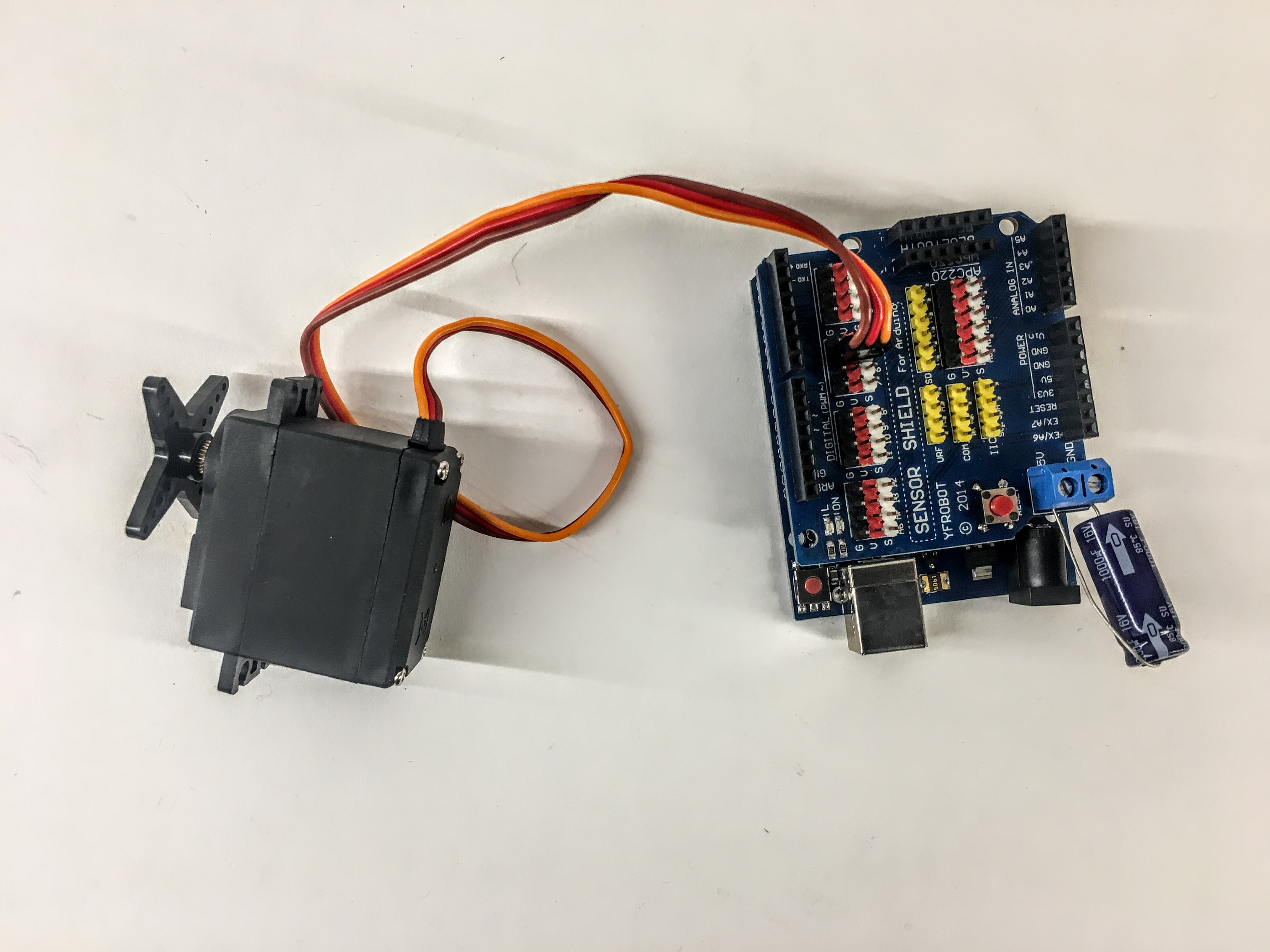``````  #include <Servo.h>

Servo myservo;  // create servo object to control a servo
// twelve servo objects can be created on most boards

int pos = 0;    // variable to store the servo position

void setup() {
myservo.attach(5);  // attaches the servo on pin 5 to the servo object
}

void loop() {

for (pos = 0; pos <= 180; pos += 1) { // goes from 0 degrees to 180 degrees
// in steps of 1 degree
myservo.write(pos);              // tell servo to go to position in variable 'pos'
delay(15);                       // waits 15ms for the servo to reach the position
}
for (pos = 180; pos >= 0; pos -= 1) { // goes from 180 degrees to 0 degrees
myservo.write(pos);              // tell servo to go to position in variable 'pos'
delay(15);                       // waits 15ms for the servo to reach the position
}
}
``````

## Timing without delay

Using delay is bad practice because it halts everything on the board. Instead use "millis()". This example does something every two seconds:

``````unsigned long timer = 0;
void setup()
{

}

void loop()
{
if(millis()-timer > 2000) // do something every two seconds)
{
timer = millis();
//do something here
}
}
``````

For the advanced student, there are more general guides on programming here and here.